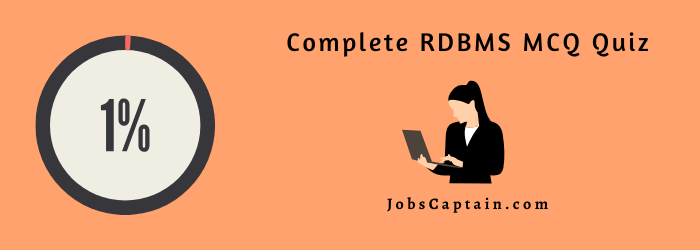## RDBMS MCQ Quiz

Question 1: A relational database consists of a collection of _________ .

(A) Tables

(B) Fields

(C) Records

(D) Keys

(A) Tables

Question 2: A ___________ in a table represents a relationship among a set of values.

(A) Column

(B) Key

(C) Row

(D) Entry

(C) Row

Question 3: Which term is used to refer to a row?

(A)Attribute

(B) Tuple

(C) Field

(D) Instance

(B) Tuple

Question 4: For each attribute of a relation, there is a set of permitted values, called the __________ of that attribute.

(A) Domain

(B) Relation

(C) Set

(D) Schema

(A) Domain

Question 5: _______ is a procedural language.

(A) Domain relational calculus

(B) Relational algebra

(C) Tuple relational calculus

(D) Query language

(B) Relational algebra

Question 6: A ________ is a query that retrieves rows from more than one table or view.

(A) Start

(B) End

(C) Join

(D) None of the mentioned

(C) Join

Question 7: Which join refers to join records from the write table that have no matching key in the left table are include in the result set?

(A) Left outer join

(B) Right outer join

(C) Full outer join

(D) Half outer join

(B) Right outer join

Question 8: Which of the following creates a virtual relation for storing the query ?

(A) Function

(B) View

(C) Procedure

(D) None of the above

(B) View

Question 9: The number of attributes in relation is called as its _________ .

(A) Cardinality

(B) Degree

(C) Tuples

(D) Entity

(B) Degree

Question 10: Functional Dependencies are the types of constraints that are based on _________ .

(A) Key

(B) Superset key

(C) Key revisited

(D) None of the above

(A) Key

Question 11: ______ forms are based on the concept of functional dependency.

(A) 1NF

(B) 2NF

(C) 3NF

(D) 4NF

(C) 3NF

Question 12: Trigger are supported in ___________ .

(A) Delete

(B) Update

(C) Views

(D) All the above

(C) Views

Question 13: _______ forms has a relation that possesses data about an individual entity.

(A) 2NF

(B) 3NF

(C) 4NF

(D) 5NF

(C) 4NF

Question 14: Referential integrity is directly related to ___________ .

(A) Relation key

(B) Foreign key

(C) Primary key

(D) Candidate key

(B) Foreign key

Question 15: E-R modeling technique is _____________ .

(A) Tree structure

(B) Top-down method

(C) Bottom-up method

(D) Right-left approach

(B) Top-down method

Question 16: ER Model Comes under ___________ .

(A) Physical data Model

(B) Record based logical Model

(C) Object based logical Model

(D) None of Above

(C) Object based logical Model

Question 17: A _________ is an association among several entities.

(A) Relationship

(B) Association

(C) Set

(D) combination

(A) Relationship

Question 18: What type of join is needed when you wish to include rows that do not have matching values?

(A) Equi-join

(B) Natural join

(C) Outer join

(D) All of the above

(C) Outer join

Question 19: ____________ is NOT a type of SQL constraint.

(A) PRIMARY KEY

(B) FOREIGN KEY

(C) ALTERNATE KEY

(D) UNIQUE

(C) ALTERNATE KEY

Question 20: In a one-to-many relationship, the entity that is on the one side of the relationship is called a(n) ___________ entity.

(A) parent

(B) child

(C) Instance

(D) Subtype

(A) parent

Question 21: ____________ is a single-entity instance of one type related to many entity instances of another type?

(A) One-to-One Relationship

(B) One-to-Many Relationship

(C) Many-to-Many Relationship

(D) Composite Relationship

(B) One-to-Many Relationship

Question 22: Properties that describe the characteristics of entities are called _______________ .

(A) Entities

(B) Attributes

(C) Identifiers

(D) Relationships

(B) Attributes

Question 23: _____________ is the function of the union operation?

(A) It combines the results of any two different queries

(B) It combines the results of two different queries which have the same set of attributes in the select clause

(C) It combines the results of two different queries which have the same condition in the where clause

(D) It gives the Cartesian product of the results of any 2 queries

(B) It combines the results of two different queries which have the same set of attributes in the select clause

Question 24: In relation algebra a new term was defined by codd as __________:

(A) Relation

(B) Relation completeness

(C) Relation operation

(D) Relation selection

(B) Relation completeness

Question 25: ___________ is a unary operation.

(A) Selection operation

(B) Primitive operation

(C) Projection operation

(D) Generalized selection

(D) Generalized selection

Question 26: How many primitive operators of relation algebra as proposed by codd?

(A) 2

(B) 3

(C) 4

(D) 6

(D) 6

Question 27: ______________  is based on specifying a number of tuple variables?

(A) Tuple relation calculus

(B) Domain relational calculus

(C) Both

(D) None of above

(A) Tuple relation calculus

Question 28: ________ is a collection of tools for describing data,data relationship,data relationship and constraint.

(A) Instances

(B) schema

(C) Data model

(D) SQL

(C) Data model

Question 29: It refers to set of one or more columns that designates the __________  in a referential integrity constraint.

(A) Select key

(B) Foreign key

(C) Write key

(D) None of the above

(B) Foreign key

Question 30: In which year relational algebra became prominent after the relational model of database was published?

(A) 1969

(B) 1970

(C) 1971

(D) 1972

(B) 1970

Question 31: ______________ is used for interfacing with RDBMS.

(A) IBM

(B) SQL

(C) ANSI

(D) ORACLE

(B) SQL

Question 32: ______________  indicates the maximum number of entities that can be involved in a relationship?

(A) Minimum cardinality

(B) Maximum cardinality

(C) ERD

(D) Greater Entity Count

(B) Maximum cardinality

Question 33: ___________ is a relationship called when it is maintained between two entities.

(A) Unary

(B) Binary

(C) Ternary

(D) Quaternary

(B) Binary

Question 34: A tablespace is further broken down into ________ .

(A) Tablespace

(B) Segments

(C) Extents

(D) Blocks